# Specific requirements

In this programming project, complete the following steps:
• Select a data set and load it into Python/R.
• Build a single layer neural networks to analyze the data of your own choosing.
• Submit a *.html file generated by Jupyter Notebook/R Markdown.

# Data frame information

### Description

Dataset of Social media ads describing users, whether users have purchased a product by clicking on the advertisements shown to them.

### Content

Social media ads of 400 rows and 5 columns.

#### User ID

Character field which catpures the user account number.

#### Gender

Character field which indicates whether the user is female or male.

#### Age

The age of the user.

#### Estimated Salary

The estimated salary of the user.

#### Purchased

A binary field which indicates whether the user made the purchase.

# Clearing R Studio Memory Usage

gc()
##           used (Mb) gc trigger (Mb) max used (Mb)
## Ncells  543103 29.1    1241057 66.3   621331 33.2
## Vcells 1025152  7.9    8388608 64.0  1600889 12.3
rm(list = ls())

# Time Counter Start

start_time <- Sys.time()

# Include the knitr package for integration of R code into Markdown

knitr::opts_chunk$set(echo = TRUE) # All the libraries used in this code library(easypackages) libraries("caret","caretEnsemble","caTools","class","cluster","data.tree","devtools","doSNOW","dplyr","e1071","factoextra","gbm","FNN","FSelector","ggalt","ggforce","ggfortify","ggplot2","gmodels","klaR","lattice","mlbench","modeest","nnet","neuralnet","outliers","parallel","psych","purrr","readr","rpart","rpart.plot","spatialEco","stats","tidyr","randomForest","ROSE","rsample","ROCR","pROC","glmnet","gridExtra","R6") # Import data oldw <- getOption("warn") options(warn = -1) library(readr) input_data <- read_csv("Social_Network_Ads.csv", col_types = cols( Age = col_number(), EstimatedSalary = col_number(), Gender = col_character(), Purchased = col_character(), User ID = col_character() ) ) options(warn = -1)  # Numeric/character field separator num.names <- input_data %>% select_if(is.numeric) %>% colnames() ch.names <- input_data %>% select_if(is.character) %>% colnames() # Descriptive statistics before data processing ### Dimension of data frame dim(input_data) ##  400 5 ### Structure of data frame str(input_data) ## tibble [400 x 5] (S3: spec_tbl_df/tbl_df/tbl/data.frame) ##$ User ID        : chr [1:400] "15624510" "15810944" "15668575" "15603246" ...
##  $Gender : chr [1:400] "Male" "Male" "Female" "Female" ... ##$ Age            : num [1:400] 19 35 26 27 19 27 27 32 25 35 ...
##  $EstimatedSalary: num [1:400] 19000 20000 43000 57000 76000 58000 84000 150000 33000 65000 ... ##$ Purchased      : chr [1:400] "0" "0" "0" "0" ...
##  - attr(*, "spec")=
##   .. cols(
##   ..   User ID = col_character(),
##   ..   Gender = col_character(),
##   ..   Age = col_number(),
##   ..   EstimatedSalary = col_number(),
##   ..   Purchased = col_character()
##   .. )

### Summary statistics of data frame

summary(input_data)
##    User ID             Gender               Age        EstimatedSalary
##  Length:400         Length:400         Min.   :18.00   Min.   : 15000
##  Class :character   Class :character   1st Qu.:29.75   1st Qu.: 43000
##  Mode  :character   Mode  :character   Median :37.00   Median : 70000
##                                        Mean   :37.66   Mean   : 69743
##                                        3rd Qu.:46.00   3rd Qu.: 88000
##                                        Max.   :60.00   Max.   :150000
##   Purchased
##  Length:400
##  Class :character
##  Mode  :character
##
##
## 

### Glimpse of data frame

glimpse(input_data)
## Rows: 400
## Columns: 5
## $User ID <chr> "15624510", "15810944", "15668575", "15603246", "15... ##$ Gender          <chr> "Male", "Male", "Female", "Female", "Male", "Male",...
## $Age <dbl> 19, 35, 26, 27, 19, 27, 27, 32, 25, 35, 26, 26, 20,... ##$ EstimatedSalary <dbl> 19000, 20000, 43000, 57000, 76000, 58000, 84000, 15...
##  37.655
##
##  37
##
##  35
##
##  18
##
##  60
##
##  18 60
##
##  109.8907
##
##  10.48288
##
##  11.8608
##
## $EstimatedSalary ##  31134.6 # Data Processing ## Converting character variables into factors ### Explanation To ensure that R’s data science models work correctly, all categorical dependent variables must be explicitly converted into factors. As for the independent variables, if the variable is both categorical and has more than two levels, then it should be converted into a factor. input_data <- as.data.frame(lapply(input_data, function(x) if(is.character(x)){ x=as.factor(x) } else x)) ## Sorting data set ### Explanation Useful for examinating the data values. By sorting the data, one can tell if there are missing or corrupted data values. input_data <- input_data[order(input_data[,1]),] glimpse(input_data) ## Rows: 400 ## Columns: 5 ##$ User.ID         <fct> 15566689, 15569641, 15570769, 15570932, 15571059, 1...
## $Gender <fct> Female, Female, Female, Male, Female, Female, Male,... ##$ Age             <dbl> 35, 58, 26, 34, 33, 21, 40, 35, 58, 35, 48, 35, 41,...
## $EstimatedSalary <dbl> 57000, 95000, 80000, 115000, 41000, 16000, 71000, 5... ##$ Purchased       <fct> 0, 1, 0, 0, 0, 0, 1, 0, 1, 0, 1, 0, 0, 0, 1, 1, 0, ...
input_data <- input_data[order(-input_data[,1]),]
glimpse(input_data)
## Rows: 400
## Columns: 5
## $User.ID <fct> 15566689, 15569641, 15570769, 15570932, 15571059, 1... ##$ Gender          <fct> Female, Female, Female, Male, Female, Female, Male,...
## $Age <dbl> 35, 58, 26, 34, 33, 21, 40, 35, 58, 35, 48, 35, 41,... ##$ EstimatedSalary <dbl> 57000, 95000, 80000, 115000, 41000, 16000, 71000, 5...
## $Purchased <fct> 0, 1, 0, 0, 0, 0, 1, 0, 1, 0, 1, 0, 0, 0, 1, 1, 0, ... ## Replacing missing values with mean or NA ### Explanation These missing values could cause inaccuracies or errors when calculating data limits, central tendency, dispersion tendency, correlation, multicollinearity, p-values, z-scores, variance inflation factors, etc. input_data <- as.data.frame(lapply(input_data, function(x) if(is.numeric(x) && is.na(x)){ mean(x, na.rm = TRUE) } else { if(is.character(x) && is.na(x)){x = "NA"} else x } )) glimpse(input_data) ## Rows: 400 ## Columns: 5 ##$ User.ID         <fct> 15566689, 15569641, 15570769, 15570932, 15571059, 1...
## $Gender <fct> Female, Female, Female, Male, Female, Female, Male,... ##$ Age             <dbl> 35, 58, 26, 34, 33, 21, 40, 35, 58, 35, 48, 35, 41,...
## $EstimatedSalary <dbl> 57000, 95000, 80000, 115000, 41000, 16000, 71000, 5... ##$ Purchased       <fct> 0, 1, 0, 0, 0, 0, 1, 0, 1, 0, 1, 0, 0, 0, 1, 1, 0, ...

## Scaling numeric variables

### Explanation

These numeric variables are centered and standardized between -1 and 1. In order to correctly calculate the distances between data points, the values of each variable have to be on the same scale. Also, it is easier to fit smaller numbers onto the axes of a graph.

#### Function for standardizing data values

Standardization = (x - mean(x))/std(x)

input_data <- as.data.frame(lapply(input_data, function(x) if(is.numeric(x)){
(x - mean(x)) / sd(x)
} else x))

str(input_data)
## 'data.frame':    400 obs. of  5 variables:
##  $User.ID : Factor w/ 400 levels "15566689","15569641",..: 1 2 3 4 5 6 7 8 9 10 ... ##$ Gender         : Factor w/ 2 levels "Female","Male": 1 1 1 2 1 1 2 2 1 1 ...
##  $Age : num -0.253 1.941 -1.112 -0.349 -0.444 ... ##$ EstimatedSalary: num  -0.374 0.741 0.301 1.327 -0.843 ...
##  $Purchased : Factor w/ 2 levels "0","1": 1 2 1 1 1 1 2 1 2 1 ... glimpse(input_data) ## Rows: 400 ## Columns: 5 ##$ User.ID         <fct> 15566689, 15569641, 15570769, 15570932, 15571059, 1...
## $Gender <fct> Female, Female, Female, Male, Female, Female, Male,... ##$ Age             <dbl> -0.25327018, 1.94078408, -1.11181315, -0.34866384, ...
## $EstimatedSalary <dbl> -0.37371367, 0.74075518, 0.30083327, 1.32731773, -0... ##$ Purchased       <fct> 0, 1, 0, 0, 0, 0, 1, 0, 1, 0, 1, 0, 0, 0, 1, 1, 0, ...

# Descriptive statistics after data processing

### Dimension of data frame

dim(input_data)
##  400   5

### Structure of data frame

str(input_data)
## 'data.frame':    400 obs. of  5 variables:
##  $User.ID : Factor w/ 400 levels "15566689","15569641",..: 1 2 3 4 5 6 7 8 9 10 ... ##$ Gender         : Factor w/ 2 levels "Female","Male": 1 1 1 2 1 1 2 2 1 1 ...
##  $Age : num -0.253 1.941 -1.112 -0.349 -0.444 ... ##$ EstimatedSalary: num  -0.374 0.741 0.301 1.327 -0.843 ...
##  $Purchased : Factor w/ 2 levels "0","1": 1 2 1 1 1 1 2 1 2 1 ... ### Summary statistics of data frame summary(input_data) ## User.ID Gender Age EstimatedSalary Purchased ## 15566689: 1 Female:204 Min. :-1.87496 Min. :-1.605495 0:257 ## 15569641: 1 Male :196 1st Qu.:-0.75409 1st Qu.:-0.784308 1:143 ## 15570769: 1 Median :-0.06248 Median : 0.007552 ## 15570932: 1 Mean : 0.00000 Mean : 0.000000 ## 15571059: 1 3rd Qu.: 0.79606 3rd Qu.: 0.535458 ## 15573452: 1 Max. : 2.13157 Max. : 2.353802 ## (Other) :394 ### Glimpse of data frame glimpse(input_data) ## Rows: 400 ## Columns: 5 ##$ User.ID         <fct> 15566689, 15569641, 15570769, 15570932, 15571059, 1...
## $Gender <fct> Female, Female, Female, Male, Female, Female, Male,... ##$ Age             <dbl> -0.25327018, 1.94078408, -1.11181315, -0.34866384, ...
## $EstimatedSalary <dbl> -0.37371367, 0.74075518, 0.30083327, 1.32731773, -0... ##$ Purchased       <fct> 0, 1, 0, 0, 0, 0, 1, 0, 1, 0, 1, 0, 0, 0, 1, 1, 0, ...

head(input_data)
##    User.ID Gender        Age EstimatedSalary Purchased
## 1 15566689 Female -0.2532702      -0.3737137         0
## 2 15569641 Female  1.9407841       0.7407552         1
## 3 15570769 Female -1.1118131       0.3008333         0
## 4 15570932   Male -0.3486638       1.3273177         0
## 5 15571059 Female -0.4440575      -0.8429637         0
## 6 15573452 Female -1.5887815      -1.5761669         0

### Tail of data frame

tail(input_data)
##      User.ID Gender        Age EstimatedSalary Purchased
## 395 15811613 Female -0.1578765       0.1541926         0
## 396 15813113   Male  0.2236981       1.0926927         1
## 397 15814004   Male -1.0164195      -1.4588544         0
## 398 15814553   Male  1.8453904      -0.2857293         1
## 399 15814816   Male -0.6348448      -0.1097605         0
## 400 15815236 Female  0.7006665       1.7965678         1

### Variable data types

sapply(input_data,mode)
##         User.ID          Gender             Age EstimatedSalary       Purchased
##       "numeric"       "numeric"       "numeric"       "numeric"       "numeric"

### Mean of each variable

lapply(input_data[,num.names],mean)
## $Age ##  -1.050681e-16 ## ##$EstimatedSalary
##  6.539365e-18

### Median of each variable

lapply(input_data[,num.names],median)
## $Age ##  -0.06248285 ## ##$EstimatedSalary
##  0.007551993

### Mode of each variable

lapply(input_data[,num.names],mfv)
## $Age ##  -0.2532702 ## ##$EstimatedSalary
##  0.06620825

### Minimum value of each variable

lapply(input_data[,num.names],min)
## $Age ##  -1.874962 ## ##$EstimatedSalary
##  -1.605495

### Maximum value of each variable

lapply(input_data[,num.names],max)
## $Age ##  2.131571 ## ##$EstimatedSalary
##  2.353802

### Range of each variable

lapply(input_data[,num.names],range)
## $Age ##  -1.874962 2.131571 ## ##$EstimatedSalary
##  -1.605495  2.353802

### Variance of each variable

lapply(input_data[,num.names],var)
## $Age ##  1 ## ##$EstimatedSalary
##  1

### Standard deviation of each variable

lapply(input_data[,num.names],sd)
## $Age ##  1 ## ##$EstimatedSalary
##  1

### Median absolute deviation of each variable

lapply(input_data[,num.names],mad)
## $Age ##  1.131445 ## ##$EstimatedSalary
##  0.9131195

# Plots and Graphs

## Box plots

### Explanation

This box plot reveals the mean value, minimum value, and maximum value of each variable.

### Observation

It appears that both Age and Estimated Salary are pretty much centered at 0. However, if it is broken down by the factor level of the target variable Purchased, each predictor variable appears to have a higher mean for transactions in which the customers did make a purchase.

oldw <- getOption("warn")
options(warn = -1)
boxplot(input_data[,num.names])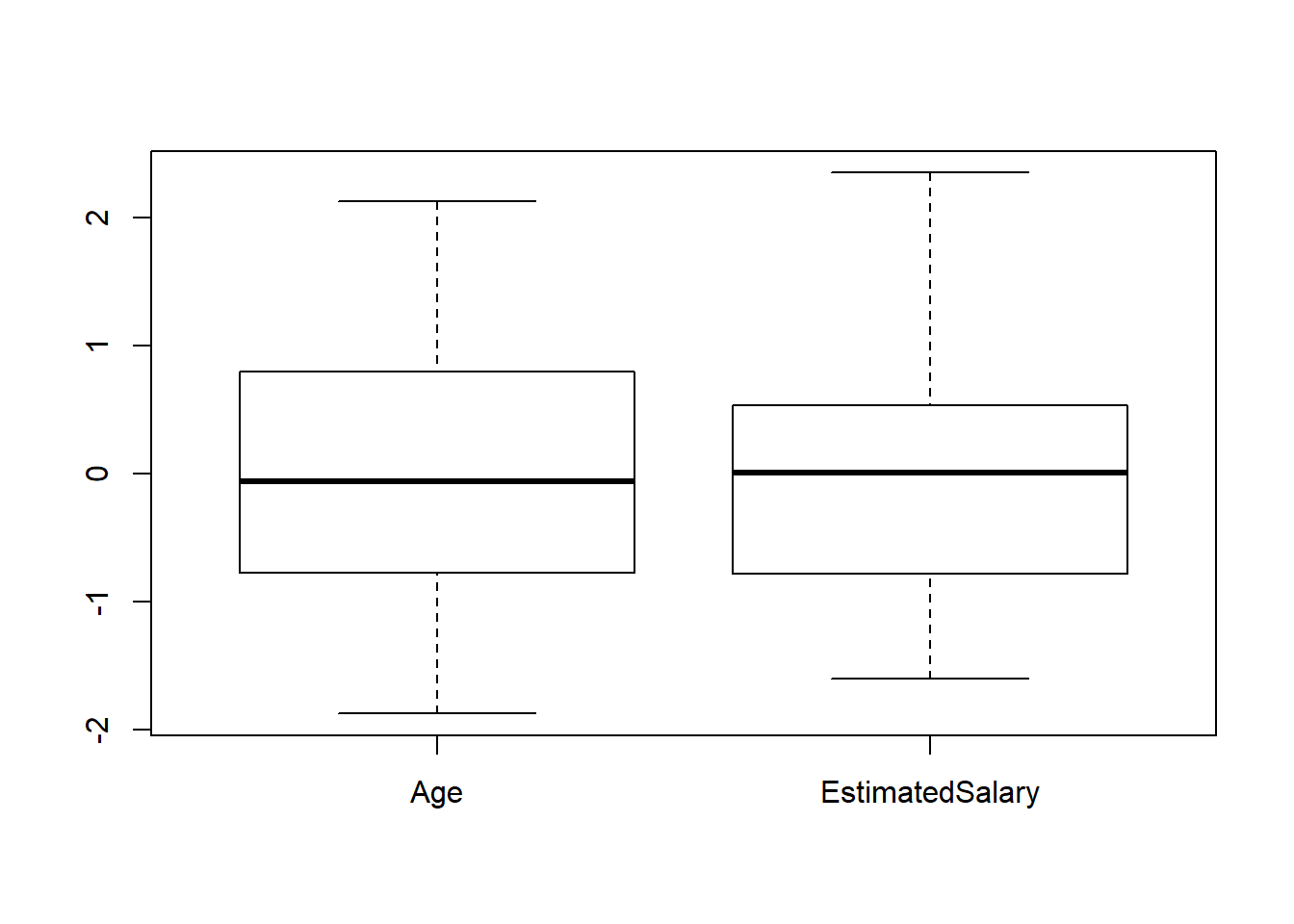options(warn = oldw)
oldw <- getOption("warn")
options(warn = -1)
ggplot(data = input_data, aes(y=Age)) + geom_boxplot(aes(fill=Purchased))+ggtitle("Box Plot of Age")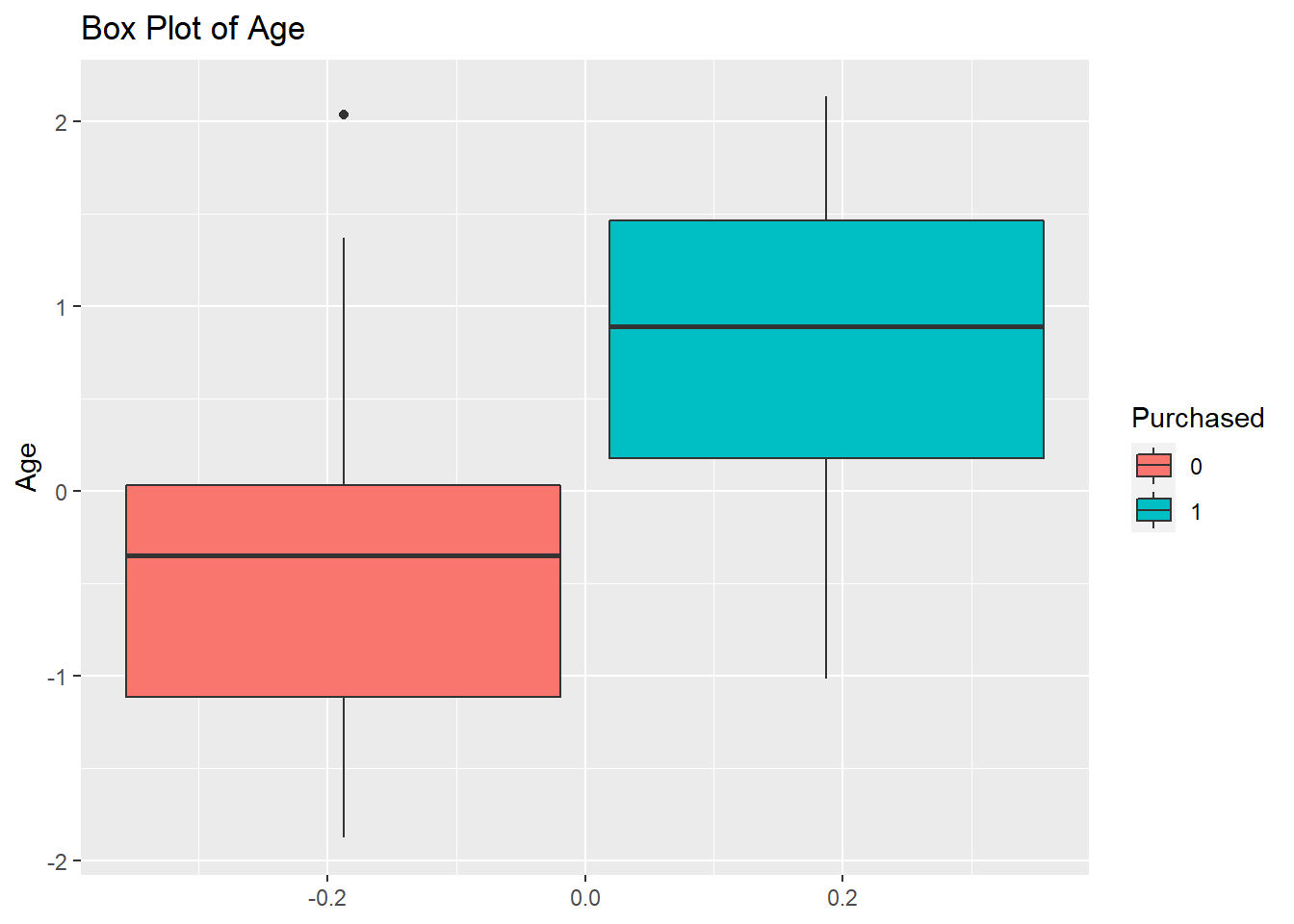ggplot(data = input_data, aes(y=EstimatedSalary)) + geom_boxplot(aes(fill=Purchased))+ggtitle("Box Plot of Estimated Salary")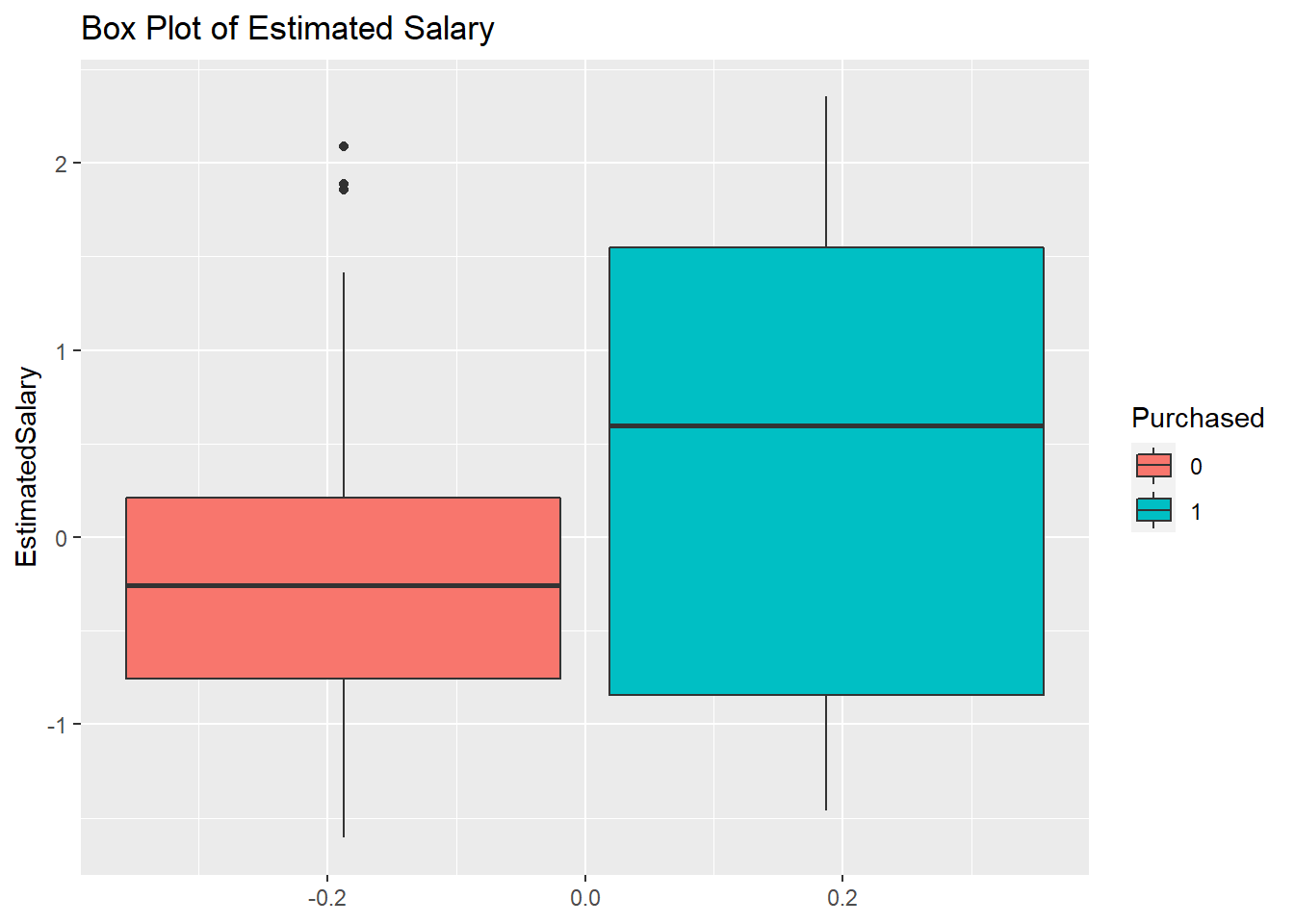options(warn = oldw)

## Histograms

### Explanation

The purpose of these histograms is to see the frequency of each predictor variable under each factor level of the target variable.

### Observation

It appears that the majority of the purchases were made by customers who have low salaries.

oldw <- getOption("warn")
options(warn = -1)
hist(input_data$Age, main = "Histogram of Age", xlab="Age")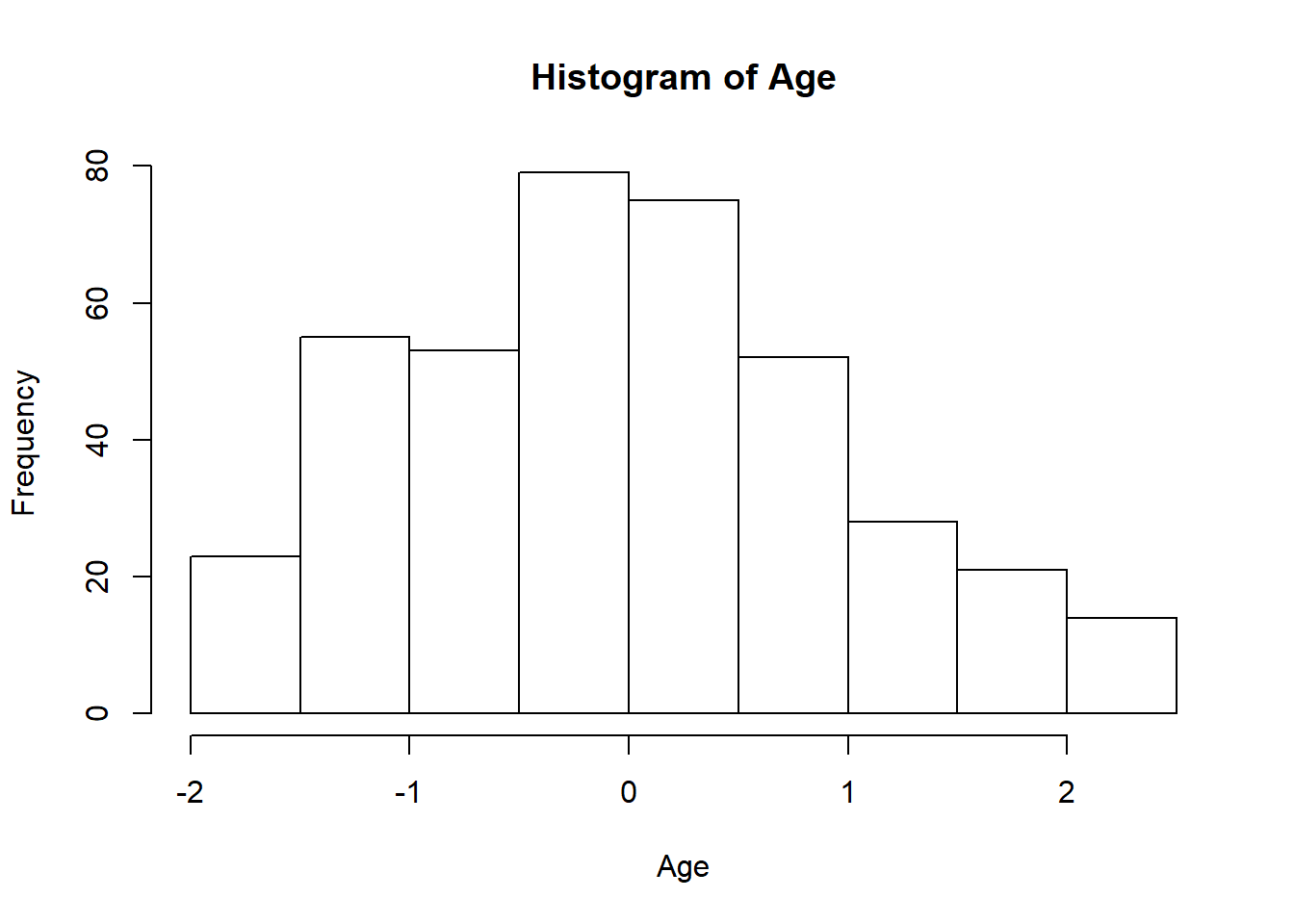hist(input_data$EstimatedSalary, main = "Histogram of Estimated Salary", xlab="Estimated Salary")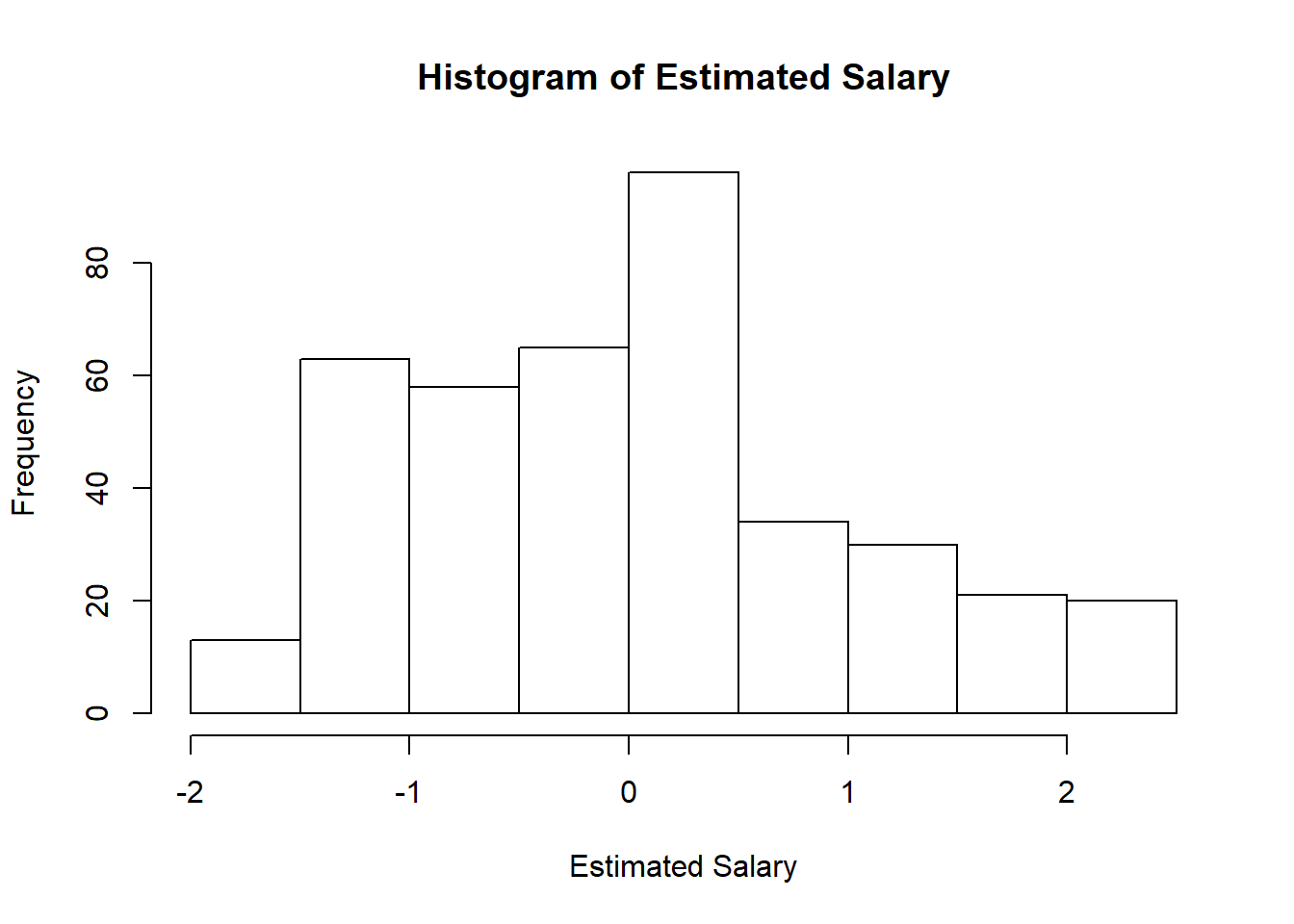options(warn = oldw)
oldw <- getOption("warn")
options(warn = -1)
ggplot(data = input_data, aes(x=Age, fill=Purchased, color=Purchased)) + geom_histogram(alpha=0.6)+ggtitle("Histogram of Age")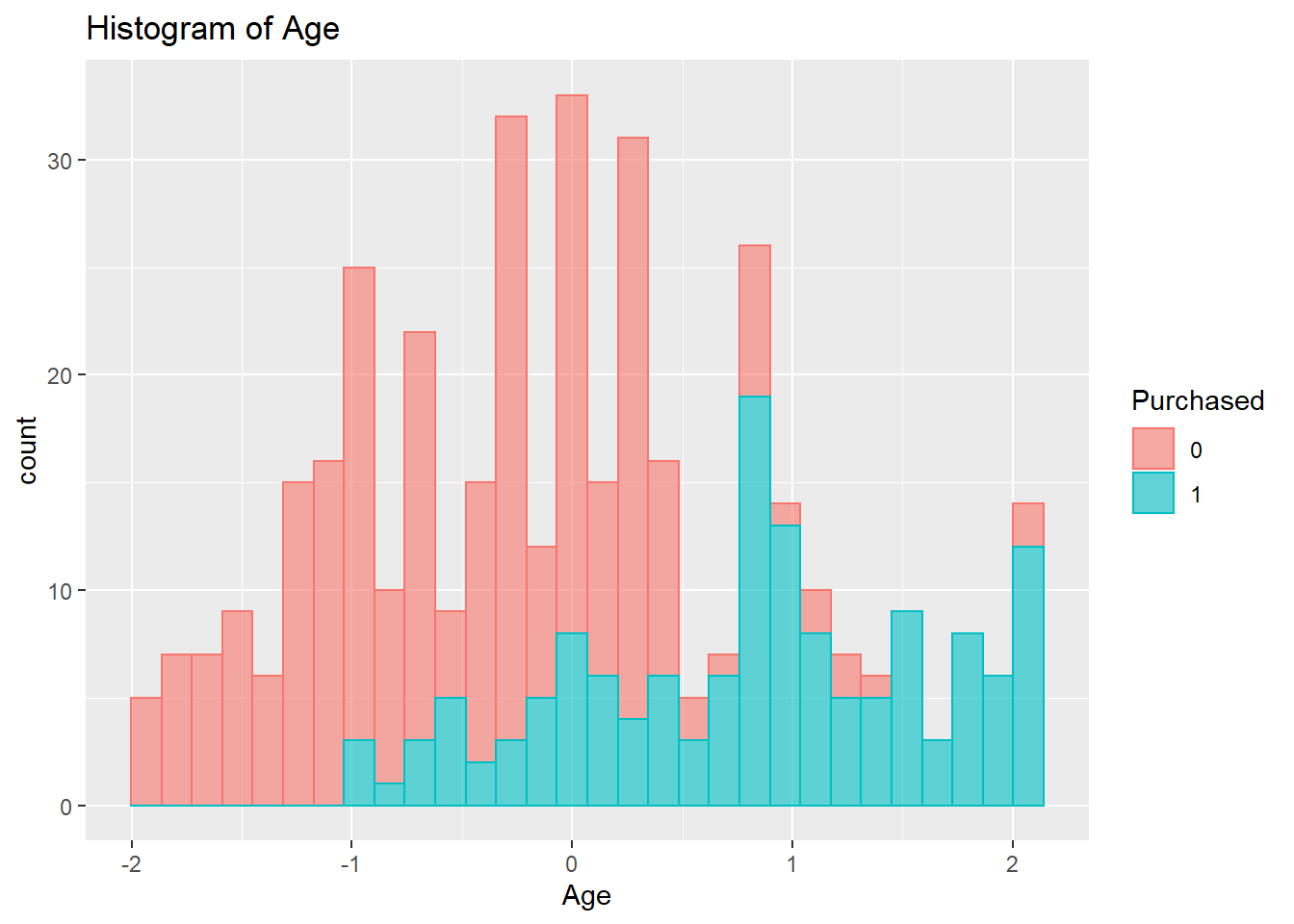ggplot(data = input_data, aes(x=EstimatedSalary, fill=Purchased, color=Purchased)) + geom_histogram(alpha=0.6)+ggtitle("Histogram of Estimated Salary")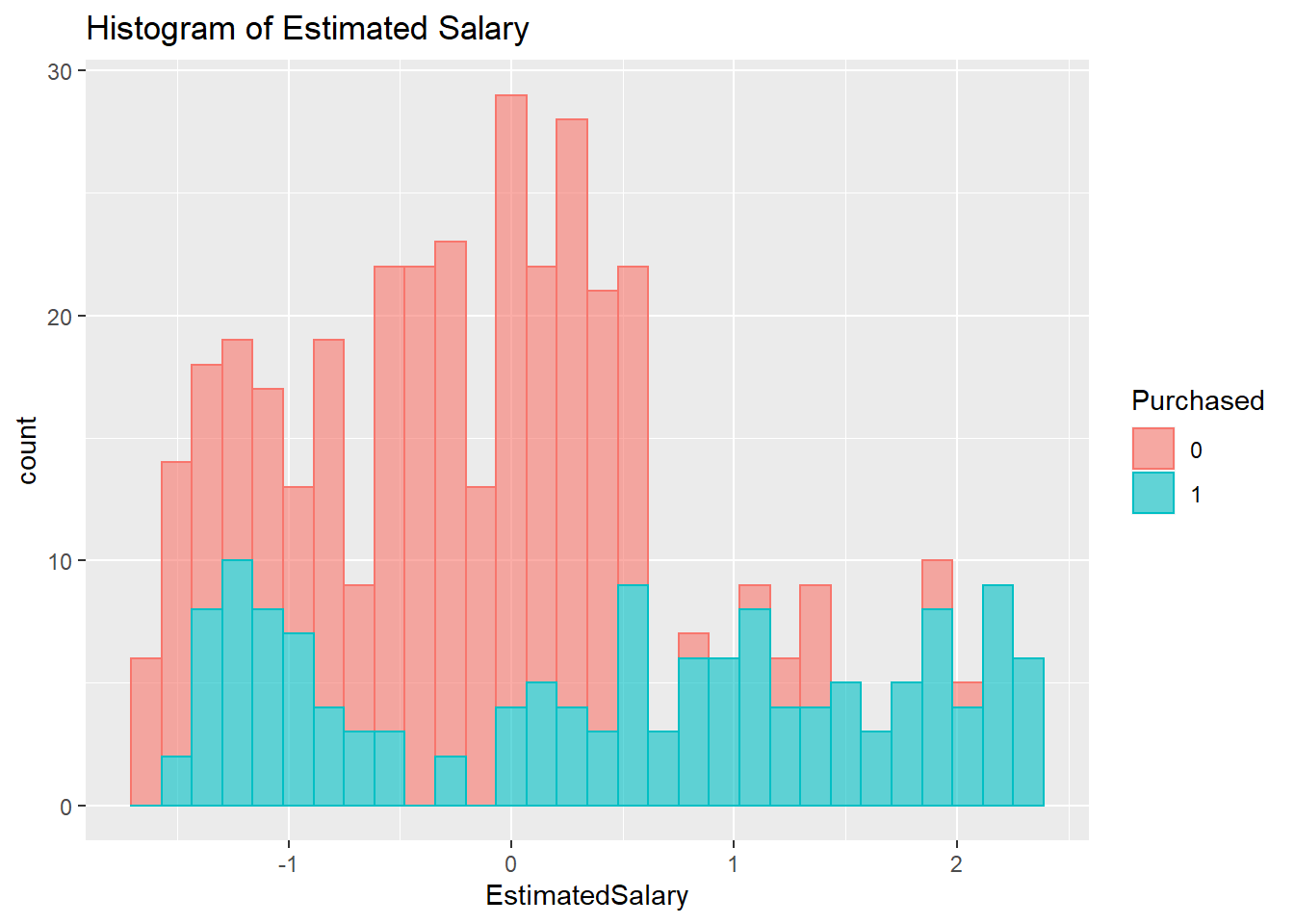options(warn = oldw)

# Grubbs’s Outlier Test

## Explanation

This is a test to check for the existence of outliers associated with each independent variable in the data frame. This test is based on Z-Scores. The function’s null hypothesis is that there are no outliers. If the p-value is smaller than 0.05, then the null hypothesis could be rejected, and the alternative hypothesis that there is at least one outlier could be accepted. The two-tail test is carried out for this data frame.

## Observation

All variables have p-values smaller than 0.05. Given a significant cut-off point of 0.05, all these variables have outliers.

# Detect outliers via z-score
grubbs.test(input_data$Age,two.sided=TRUE,type=11) ## ## Grubbs test for two opposite outliers ## ## data: input_data$Age
## G = 4.0065, U = 0.9798, p-value < 2.2e-16
## alternative hypothesis: -1.87496245115082 and 2.1315714052895 are outliers
grubbs.test(input_data$EstimatedSalary,two.sided=TRUE,type=11) ## ## Grubbs test for two opposite outliers ## ## data: input_data$EstimatedSalary
## G = 3.95930, U = 0.97965, p-value < 2.2e-16
## alternative hypothesis: -1.60549502203623 and 2.35380219630219 are outliers

# Correlation Analysis

## Explanation

The correlation statistics reveal the degree of associations between variables in the data set. Given a range between 0 and 1, a correlation value less than 0.5 in either direction indicates a weak correlation, and a value equal to or greater than 0.5 in either direction indicates a moderate to strong correlation.

## Observation

It appears that none of the variables have correlation coefficient greater than 0.5.

# Correlation Plot

oldw <- getOption("warn")
options(warn = -1)
pairs.panels(input_data[,num.names],gap=0,bg=c("green","red","yellow","blue","pink","purple"),pch= 21, cex=0.5)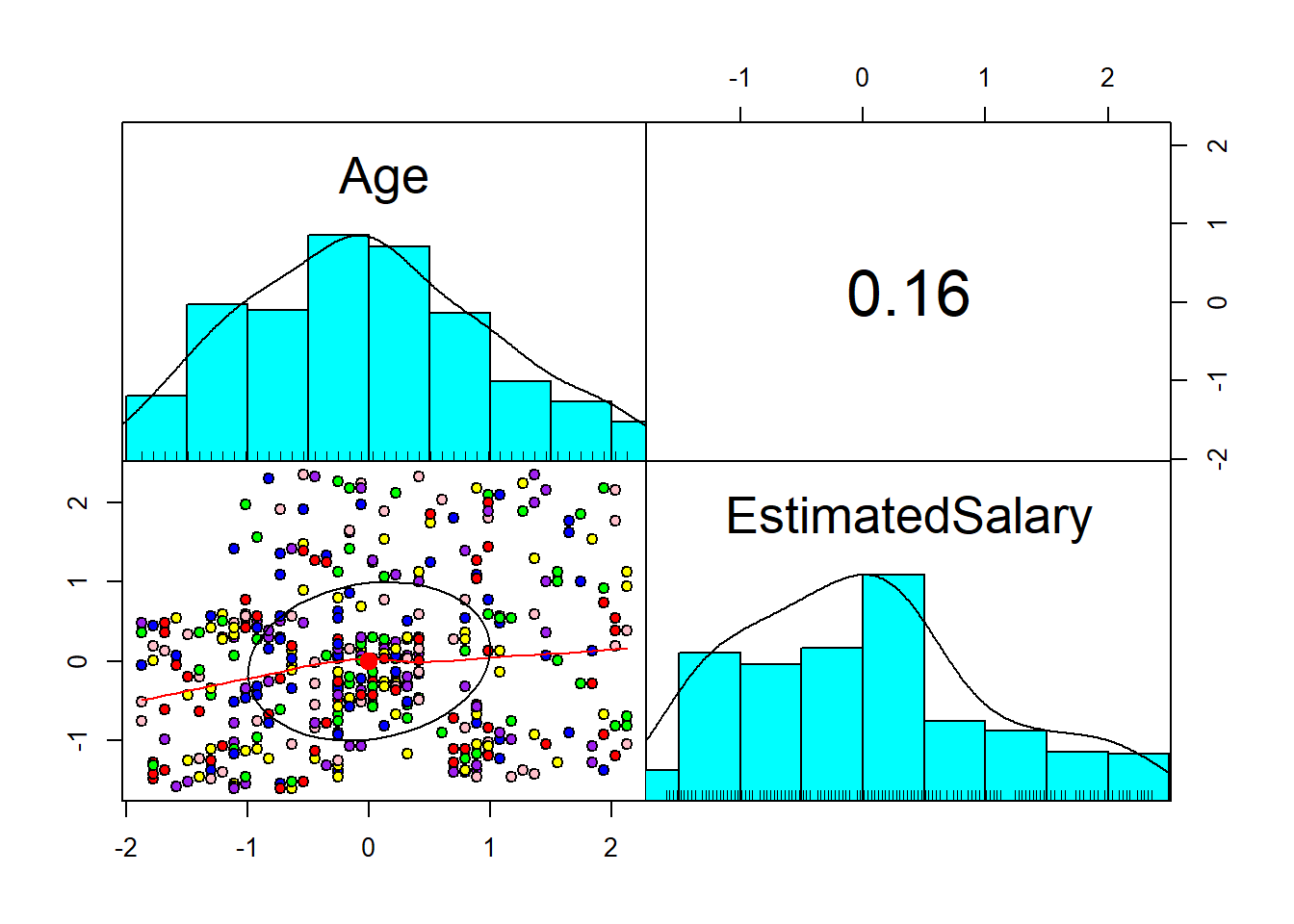options(warn = oldw)

# Correlation Table

oldw <- getOption("warn")
options(warn = -1)
cor(input_data[,num.names])
##                      Age EstimatedSalary
## Age             1.000000        0.155238
## EstimatedSalary 0.155238        1.000000
options(warn = oldw)

# Preparation of Training and Test Data Sets

## Explanation

The purpose of creating separate data sets for training and testing the model is because we want to see how differently the model would perform with data that it has never seen before.

oldw <- getOption("warn")
options(warn = -1)

set.seed(123)
df <- input_data[,c(3,4,5)]
ind <- sample(2, nrow(input_data), replace=T, prob=c(0.6,0.4))
df_sample_train <- df[ind==1,]
df_sample_test <- df[ind==2,]
options(warn = oldw)

# Single-Layer Perceptrons Neural Network

## Explanation

As the “neural” part of their name suggests, they are brain-inspired systems which are intended to replicate the way that we humans learn. Neural networks consist of input and output layers, as well as (in most cases) a hidden layer consisting of neurons that transform the input into something that the output layer can use. In case of a single-layer perceptrons, there is no hidden layer. They are excellent tools for finding patterns which are far too complex or numerous for a human programmer to extract and teach the machine to recognize.

## Interpretation

After training the model with 100 repeats and layers of 5 neurons each, the model predictively ability reached 81.4% accuracy, 83.64% sensitivity, 80% specificity, and a Kappa statistic of 0.61. An accuracy percentage of 81.4% means that the model can predict 81.4% of both the true negatives and true positives. A sensitivity of 83.64% means that the model can predict 83.64% of the true positives. A specificity percentage of 80% means that the model can predict 80% of the true negatives. A Kappa statistic of 0.61 means that the instances classified by the model matched the output labels 61% of the time. If compared to the Raw Implementation, Neuralnet has a slightly lower accuracy percentage.

oldw <- getOption("warn")
options(warn = -1)

# Making sure the target variable is in numeric format
df_sample_train$Purchased = as.integer(as.character(df_sample_train$Purchased))
df_sample_test$Purchased = as.integer(as.character(df_sample_test$Purchased))

# neuralnet cannot accept y~. as formula
nn_train <- neuralnet(formula=Purchased~Age+EstimatedSalary, data=df_sample_train, hidden=0, act.fct = "logistic", linear.output=FALSE, rep=100) #linear.output=FALSE is neccessary for classifcation NN

# Neural Network Result
head(as.data.frame(nn_train$result.matrix), n=5) ## V1 V2 V3 V4 ## error 11.059271306 11.059077415 11.059273842 11.059229745 ## reached.threshold 0.009878695 0.009526415 0.008802204 0.007929586 ## steps 72.000000000 116.000000000 107.000000000 85.000000000 ## Intercept.to.Purchased -1.006527757 -1.011272341 -1.007888882 -1.007248311 ## Age.to.Purchased 4.006363167 4.028986345 4.007153594 4.010458597 ## V5 V6 V7 V8 ## error 11.059131503 11.059176898 11.05910045 11.05931784 ## reached.threshold 0.007663115 0.008140279 0.00930926 0.00966322 ## steps 120.000000000 102.000000000 120.00000000 92.00000000 ## Intercept.to.Purchased -1.008061746 -1.008012441 -1.01078711 -1.00443101 ## Age.to.Purchased 4.019883048 4.015286049 4.02410662 4.00366746 ## V9 V10 V11 V12 ## error 11.059179190 11.059050019 11.059081449 11.059059468 ## reached.threshold 0.008646881 0.007810222 0.007006843 0.007861171 ## steps 96.000000000 111.000000000 108.000000000 107.000000000 ## Intercept.to.Purchased -1.009798139 -1.011458163 -1.008765157 -1.011095941 ## Age.to.Purchased 4.016044608 4.030347374 4.025778819 4.028973482 ## V13 V14 V15 V16 ## error 11.059127002 11.059290044 11.059255970 11.059084280 ## reached.threshold 0.008629314 0.009958285 0.007425126 0.009433234 ## steps 112.000000000 107.000000000 102.000000000 113.000000000 ## Intercept.to.Purchased -1.008292553 -1.004707178 -1.005246477 -1.011846904 ## Age.to.Purchased 4.020296384 4.006203957 4.008012917 4.026686478 ## V17 V18 V19 V20 ## error 11.059183685 11.059232360 11.05939523 11.059123561 ## reached.threshold 0.008535515 0.009924924 0.00982961 0.008416146 ## steps 105.000000000 111.000000000 86.00000000 79.000000000 ## Intercept.to.Purchased -1.007249153 -1.006193608 -1.00354962 -1.007991301 ## Age.to.Purchased 4.014390540 4.009608047 3.99641299 4.020698857 ## V21 V22 V23 V24 ## error 11.059107956 11.059250437 11.059127493 11.05926220 ## reached.threshold 0.007885998 0.009286286 0.008915809 0.00893357 ## steps 121.000000000 72.000000000 128.000000000 84.00000000 ## Intercept.to.Purchased -1.008778114 -1.005559764 -1.009618147 -1.00496661 ## Age.to.Purchased 4.022518545 4.008062405 4.020619209 4.00713336 ## V25 V26 V27 V28 ## error 11.059175740 11.059123856 11.059190724 11.059162277 ## reached.threshold 0.008977766 0.009387454 0.007896632 0.009352247 ## steps 129.000000000 115.000000000 105.000000000 103.000000000 ## Intercept.to.Purchased -1.007423130 -1.008407412 -1.007448084 -1.007310327 ## Age.to.Purchased 4.015124755 4.020663260 4.013901789 4.016473573 ## V29 V30 V31 V32 ## error 11.059140872 11.059235960 11.059133854 11.0591071 ## reached.threshold 0.008489665 0.009809328 0.008095016 0.0077181 ## steps 95.000000000 107.000000000 102.000000000 92.0000000 ## Intercept.to.Purchased -1.009790875 -1.005153554 -1.007789333 -1.0089469 ## Age.to.Purchased 4.019473280 4.009439585 4.019574344 4.0226565 ## V33 V34 V35 V36 ## error 11.059073928 11.059066837 11.059111638 11.059089444 ## reached.threshold 0.009518364 0.009080503 0.008105408 0.009073575 ## steps 120.000000000 113.000000000 116.000000000 124.000000000 ## Intercept.to.Purchased -1.010238614 -1.010669648 -1.009939504 -1.009851693 ## Age.to.Purchased 4.026935181 4.027905744 4.022498859 4.026730873 ## V37 V38 V39 V40 ## error 11.059276054 11.059054313 11.059254331 11.05913086 ## reached.threshold 0.008816792 0.008932634 0.009187266 0.00887198 ## steps 54.000000000 130.000000000 78.000000000 93.00000000 ## Intercept.to.Purchased -1.006248820 -1.011345959 -1.007836465 -1.00765028 ## Age.to.Purchased 4.006126549 4.031683647 4.008473298 4.01990797 ## V41 V42 V43 V44 ## error 11.059205989 11.059247338 11.0592275 11.059144909 ## reached.threshold 0.009200441 0.009507909 0.0091811 0.008959096 ## steps 117.000000000 107.000000000 93.0000000 96.000000000 ## Intercept.to.Purchased -1.005700066 -1.006330977 -1.0076605 -1.008868768 ## Age.to.Purchased 4.012272016 4.008352184 4.0105129 4.018531654 ## V45 V46 V47 V48 ## error 11.05921139 11.059242766 11.059201632 11.059246191 ## reached.threshold 0.00912173 0.009348176 0.009317124 0.009868915 ## steps 64.00000000 114.000000000 107.000000000 93.000000000 ## Intercept.to.Purchased -1.00491760 -1.005797868 -1.007471503 -1.004757171 ## Age.to.Purchased 4.01210141 4.008730425 4.012656237 4.008592087 ## V49 V50 V51 V52 ## error 11.059088590 11.059157952 11.059139168 11.059261879 ## reached.threshold 0.008861396 0.009942644 0.008284701 0.009738609 ## steps 133.000000000 120.000000000 126.000000000 100.000000000 ## Intercept.to.Purchased -1.010047730 -1.008739082 -1.009395624 -1.005501414 ## Age.to.Purchased 4.025021035 4.017138241 4.019430435 4.006995212 ## V53 V54 V55 V56 ## error 11.0591753 11.059156254 11.059270126 11.059125669 ## reached.threshold 0.0088874 0.007893245 0.007403058 0.008106747 ## steps 123.0000000 91.000000000 68.000000000 103.000000000 ## Intercept.to.Purchased -1.0070434 -1.007005796 -1.005872437 -1.008471847 ## Age.to.Purchased 4.0151658 4.017252139 4.007083764 4.020497573 ## V57 V58 V59 V60 ## error 11.059164471 11.059130525 11.059124230 11.059499082 ## reached.threshold 0.007942492 0.009869703 0.008022102 0.009552857 ## steps 87.000000000 108.000000000 92.000000000 78.000000000 ## Intercept.to.Purchased -1.007035919 -1.008052746 -1.007053770 -1.002765724 ## Age.to.Purchased 4.017583273 4.019958137 4.020922476 3.989445276 ## V61 V62 V63 V64 ## error 11.059150516 11.059353768 11.059162291 11.059092731 ## reached.threshold 0.009785793 0.009855779 0.009974099 0.008524386 ## steps 114.000000000 71.000000000 115.000000000 122.000000000 ## Intercept.to.Purchased -1.008014812 -1.003634544 -1.009080940 -1.009754426 ## Age.to.Purchased 4.017726739 3.999511593 4.018721663 4.024437987 ## V65 V66 V67 V68 ## error 11.059256877 11.059190496 11.059198918 11.05931855 ## reached.threshold 0.009738715 0.008999318 0.008678656 0.00959872 ## steps 112.000000000 114.000000000 105.000000000 103.00000000 ## Intercept.to.Purchased -1.006005691 -1.008494164 -1.006820358 -1.00479096 ## Age.to.Purchased 4.008971221 4.014114396 4.012879827 4.00231099 ## V69 V70 V71 V72 ## error 11.059100756 11.059214127 11.059258918 11.059078436 ## reached.threshold 0.007339124 0.009071242 0.009827834 0.009287193 ## steps 135.000000000 116.000000000 83.000000000 96.000000000 ## Intercept.to.Purchased -1.008468014 -1.005710128 -1.005368740 -1.007704454 ## Age.to.Purchased 4.023398911 4.011460883 4.007252144 4.028457519 ## V73 V74 V75 V76 ## error 11.059244271 11.05913767 11.059144002 11.059194838 ## reached.threshold 0.009669865 0.00959015 0.009495725 0.009368973 ## steps 89.000000000 98.00000000 101.000000000 98.000000000 ## Intercept.to.Purchased -1.006597594 -1.00893448 -1.008595483 -1.006273727 ## Age.to.Purchased 4.008644078 4.01925848 4.018504538 4.013242732 ## V77 V78 V79 V80 ## error 11.059216156 11.059121216 11.059114383 11.05914935 ## reached.threshold 0.009860672 0.007376398 0.009687434 0.00838519 ## steps 103.000000000 104.000000000 113.000000000 132.00000000 ## Intercept.to.Purchased -1.006790540 -1.007329814 -1.009378170 -1.00919436 ## Age.to.Purchased 4.012875154 4.021193415 4.021900737 4.01833290 ## V81 V82 V83 V84 ## error 11.059100580 11.059112829 11.059172910 11.059241979 ## reached.threshold 0.007774792 0.009745111 0.009008136 0.008861744 ## steps 110.000000000 113.000000000 114.000000000 87.000000000 ## Intercept.to.Purchased -1.009898955 -1.008686120 -1.007190100 -1.006500568 ## Age.to.Purchased 4.023685515 4.024063864 4.015391776 4.008961686 ## V85 V86 V87 V88 ## error 11.059372397 11.059169628 11.059133490 11.059187086 ## reached.threshold 0.009612282 0.009087914 0.008460542 0.009939838 ## steps 89.000000000 102.000000000 110.000000000 103.000000000 ## Intercept.to.Purchased -1.005302285 -1.007468174 -1.008204051 -1.006872383 ## Age.to.Purchased 3.998509222 4.015726700 4.019590761 4.015884598 ## V89 V90 V91 V92 ## error 11.059145678 11.059161225 11.059118063 11.059151681 ## reached.threshold 0.009395439 0.009087876 0.009929384 0.009394086 ## steps 99.000000000 87.000000000 107.000000000 112.000000000 ## Intercept.to.Purchased -1.007008656 -1.005994505 -1.009394197 -1.008183405 ## Age.to.Purchased 4.020360277 4.017091738 4.021515176 4.017625178 ## V93 V94 V95 V96 ## error 11.05917573 11.059337089 11.059067051 11.059221958 ## reached.threshold 0.00761517 0.009872248 0.007027954 0.009484249 ## steps 99.00000000 92.000000000 100.000000000 69.000000000 ## Intercept.to.Purchased -1.00578702 -1.002985662 -1.010854645 -1.006204334 ## Age.to.Purchased 4.01558741 4.001046420 4.028027010 4.010605359 ## V97 V98 V99 V100 ## error 11.05920347 11.059145541 11.059138904 11.059170688 ## reached.threshold 0.00851572 0.008946364 0.007672549 0.009795812 ## steps 89.00000000 109.000000000 120.000000000 99.000000000 ## Intercept.to.Purchased -1.00615017 -1.010049422 -1.007932043 -1.007962992 ## Age.to.Purchased 4.01248547 4.019147446 4.019085605 4.015656871 # Neural Network Plot plot(nn_train, rep="best") #rep="best" must be included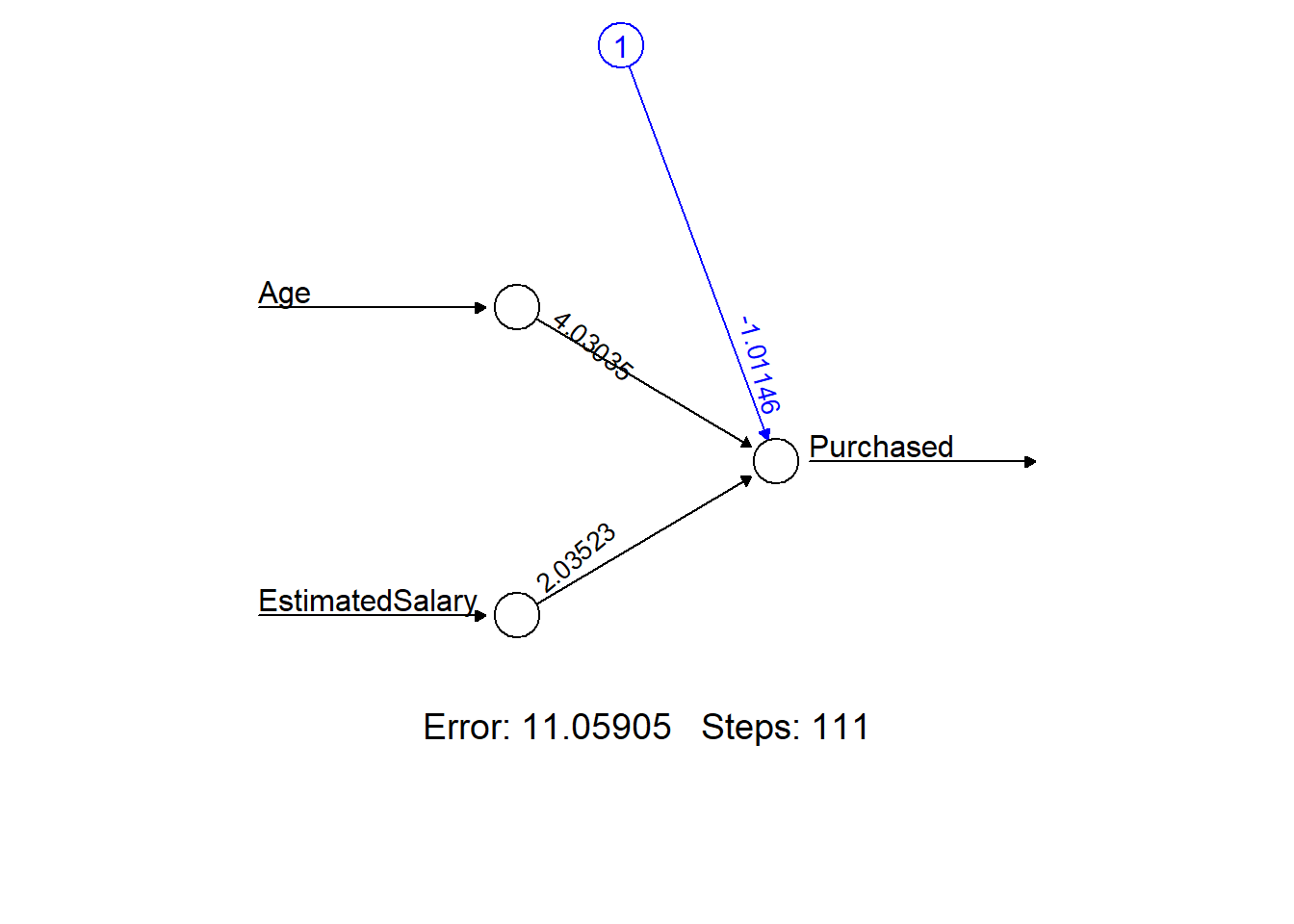# Test the neural network on some test data nn_pred <- compute(nn_train, df_sample_test) options(warn = oldw) ## Confusion Matrix ### Explanation Comparison between the predicted values and actual observed values. Calculate the average accuracy statistics. oldw <- getOption("warn") options(warn = -1) # nn_pred$net.result
pred_simo <- ifelse(nn_pred$net.result>0.5, 1, 0) # head(pred_simo, n=5) table(pred_simo) ## pred_simo ## 0 1 ## 90 66 table(df_sample_test$Purchased)
##
##   0   1
## 101  55
# table(Predicted=pred_simo,Actual=df_sample_test$Purchased) confusionMatrix( table(Predicted=pred_simo,Actual=df_sample_test$Purchased), positive='1' )
## Confusion Matrix and Statistics
##
##          Actual
## Predicted  0  1
##         0 81  9
##         1 20 46
##
##                Accuracy : 0.8141
##                  95% CI : (0.7441, 0.8718)
##     No Information Rate : 0.6474
##     P-Value [Acc > NIR] : 3.674e-06
##
##                   Kappa : 0.6105
##
##  Mcnemar's Test P-Value : 0.06332
##
##             Sensitivity : 0.8364
##             Specificity : 0.8020
##          Pos Pred Value : 0.6970
##          Neg Pred Value : 0.9000
##              Prevalence : 0.3526
##          Detection Rate : 0.2949
##    Detection Prevalence : 0.4231
##       Balanced Accuracy : 0.8192
##
##        'Positive' Class : 1
## 
options(warn = oldw)

# Single-Layer Perceptrons Neural Network

## Description

This is the raw implementation of a Single-Layer Perceptions Neural Network. This implementation is inspired by my own understanding of how a neural network works.

## Interpretation

After training the model with 10 neurons, learning rate of 0.001, and 100,000 iterations, the model was able to achieve a 95% accuracy. Since there is only one layer of neurons, there might be a problem with overfitting. However, the testing result is surprisingly good. When running the model against the test data, it was able to achieve an 83% accuracy.

oldw <- getOption("warn")
options(warn = -1)

# Making sure the target variable is in the factor format
df_sample_train$Purchased = as.factor(as.character(df_sample_train$Purchased))
df_sample_test$Purchased = as.factor(as.character(df_sample_test$Purchased))

# This functin is to convert the matrix values to a scale between -1 and 1
standardFN <- function(x) 1 / (1 + exp(-x))

# X is a matrix of predictor variable values. wgt1 and wgt2 are matrices of the assigned weights.
feedforwardNN <- function(x, wgt1, wgt2) {
# This function does both a compound multiplication and a sum product of weights+bias (wgt1,wgt2) with the input data values. At the final stage, it sum up each resulting row of products into a single column. A a vector of random bias values between 0 and 1 is used. By default, runif generates random numbers between 0 and 1.
# The cbind function adds a bias vector of 1's to the matrix. R matrix multiplication of A %*% B requires that total column number of A must match total row number of B.

set.seed(123) # Set reproduciblity for runif.
cal1 <- cbind(runif(nrow(x)), x) %*% wgt1 # Result in a matrix of n columns
calSD <- standardFN(cal1)
cal2 <- cbind(runif(nrow(calSD)), calSD) %*% wgt2 # Result in a matrix of 1 column
list(output = standardFN(cal2), cal1=cal1, cal2=cal2, calSD=calSD)
}

backpropagateNN <- function(x, y, yHAT, wgt1, wgt2, m1, learnRate) {
# Using the the differences between predicted outputs and the actual outputs to re-calcuate the weights.
# Since R matrix mulitplication requires that matrix A's number of columns must match with matrix B's number of rows, matrix A in this case has to be tranpsosed.
# y is either a 0 or 1. yHAT is a numeric value between -1 and 1.

set.seed(123) # Set reproduciblity for runif.
nm1  <- (yHAT - y) %*% t(wgt2[-1, , drop = FALSE])
nwgt1 <- t(cbind(runif(nrow(x)), x)) %*% (m1 * (1 - m1) * nm1)
nwgt2 <- t(cbind(runif(nrow(x)), m1)) %*% (yHAT - y)
wgt1 <- wgt1 - learnRate * nwgt1
wgt2 <- wgt2 - learnRate * nwgt2
list(wgt1 = wgt1, wgt2 = wgt2)
}

# Defaults of 1 neuron, learn rate of 0.01, and 10 iteration
trainNN <- function(x, y, neurons = 1, learnRate = 0.01, iterates = 10) {
set.seed(123) # Set reproducibility for rnorm.
inp <- ncol(x) + 1 # Number of inputs
wgt1 <- matrix(0.1*rnorm(inp * neurons), inp, neurons) # First initial weight matrix of 'inp' rows and 'neurons' columns
wgt2 <- as.matrix(0.1*rnorm(neurons + 1)) # Second initial weight matrix. A single-column matrix of (neurons + 1) rows.

for (i in 1:iterates) {
ff <- feedforwardNN(x, wgt1, wgt2)
bp <- backpropagateNN(x, y,
yHAT = ff$output, wgt1, wgt2, m1 = ff$calSD,
learnRate = learnRate)
wgt1 <- bp$wgt1; wgt2 <- bp$wgt2
}
list(output = ff$output, wgt1 = wgt1, wgt2 = wgt2) } x <-data.matrix(df_sample_train[,c(1, 2)]) # Matrix of inputs y <- df_sample_train$Purchased == '1' # Boolean of outputs

# Training the algorithm with 10 neurons, learn rate of 0.001, and 100,000 iterations
nnOBJ <- trainNN(x, y, neurons = 10, learnRate = 0.001, iterates = 1e5)

# Model Accuracy Percentage
mean((nnOBJ$output > .5) == y) ##  0.9467213 # Plotting the training result gridTrainBG <- expand.grid(x1 = seq(min(df_sample_train$Age)-1,
max(df_sample_train$Age)+1, by = .03), x2 = seq(min(df_sample_train$EstimatedSalary)-1,
max(df_sample_train$EstimatedSalary)+1, by = .03)) gridTrainOBJ <- feedforwardNN(x = data.matrix(gridTrainBG[, c('x1', 'x2')]), wgt1 = nnOBJ$wgt1, wgt2 = nnOBJ$wgt2) gridTrainBG$class <- factor( (gridTrainOBJ$output > .5) * 1, labels = levels(df_sample_train$Purchased) )

ggplot() +
geom_point(data = gridTrainBG, aes(x=x1, y=x2, colour = class), size = .25) +
geom_point(data = df_sample_train, aes(x=Age, y=EstimatedSalary, colour = Purchased)) +
labs(x = 'Age', y = 'Estimated Salary') +
ggtitle("Single-Layer Neural Network Training Result")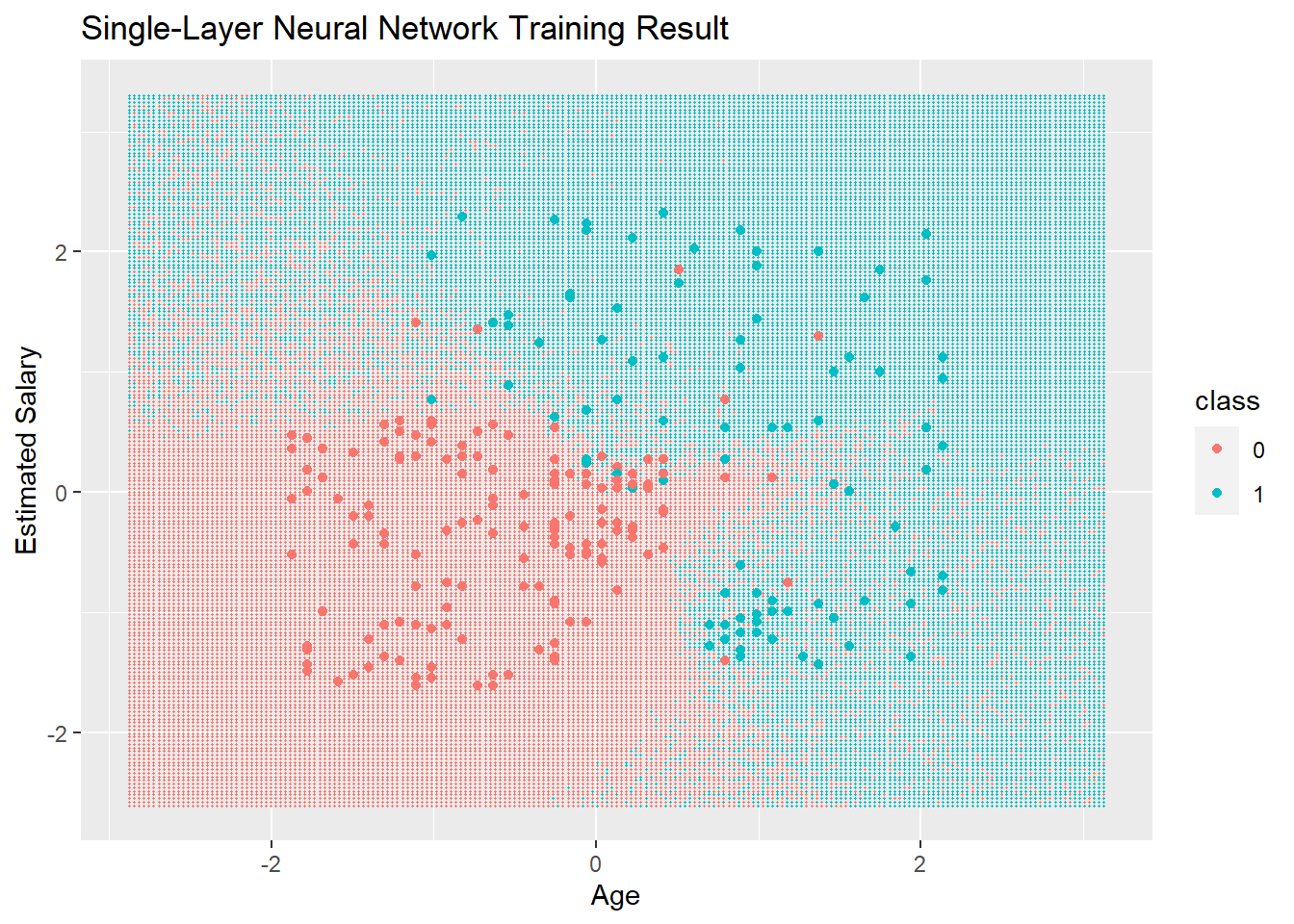# Pediction Accuracy Percentage
y <- df_sample_test$Purchased == '1' # Boolean of outputs testOBJ <- feedforwardNN(x = data.matrix(df_sample_test[,1:2]), wgt1 = nnOBJ$wgt1, wgt2 = nnOBJ$wgt2) mean((testOBJ$output > .5) == y)
##  0.8269231
# Plotting the testing result
gridTestBG <- expand.grid(x1 = seq(min(df_sample_test$Age)-1, max(df_sample_test$Age)+1,
by = .03),
x2 = seq(min(df_sample_test$EstimatedSalary)-1, max(df_sample_test$EstimatedSalary)+1,
by = .03))

gridTestOBJ <- feedforwardNN(x = data.matrix(gridTestBG[, c('x1', 'x2')]), wgt1 = nnOBJ$wgt1, wgt2 = nnOBJ$wgt2)
gridTestBG$class <- factor( (gridTestOBJ$output > .5) * 1, labels = levels(df_sample_test\$Purchased) )

ggplot() +
geom_point(data = gridTestBG, aes(x=x1, y=x2, colour = class), size = .25) +
geom_point(data = df_sample_test, aes(x=Age, y=EstimatedSalary, colour = Purchased)) +
labs(x = 'Age', y = 'Estimated Salary') +
ggtitle("Single-Layer Neural Network Testing Result")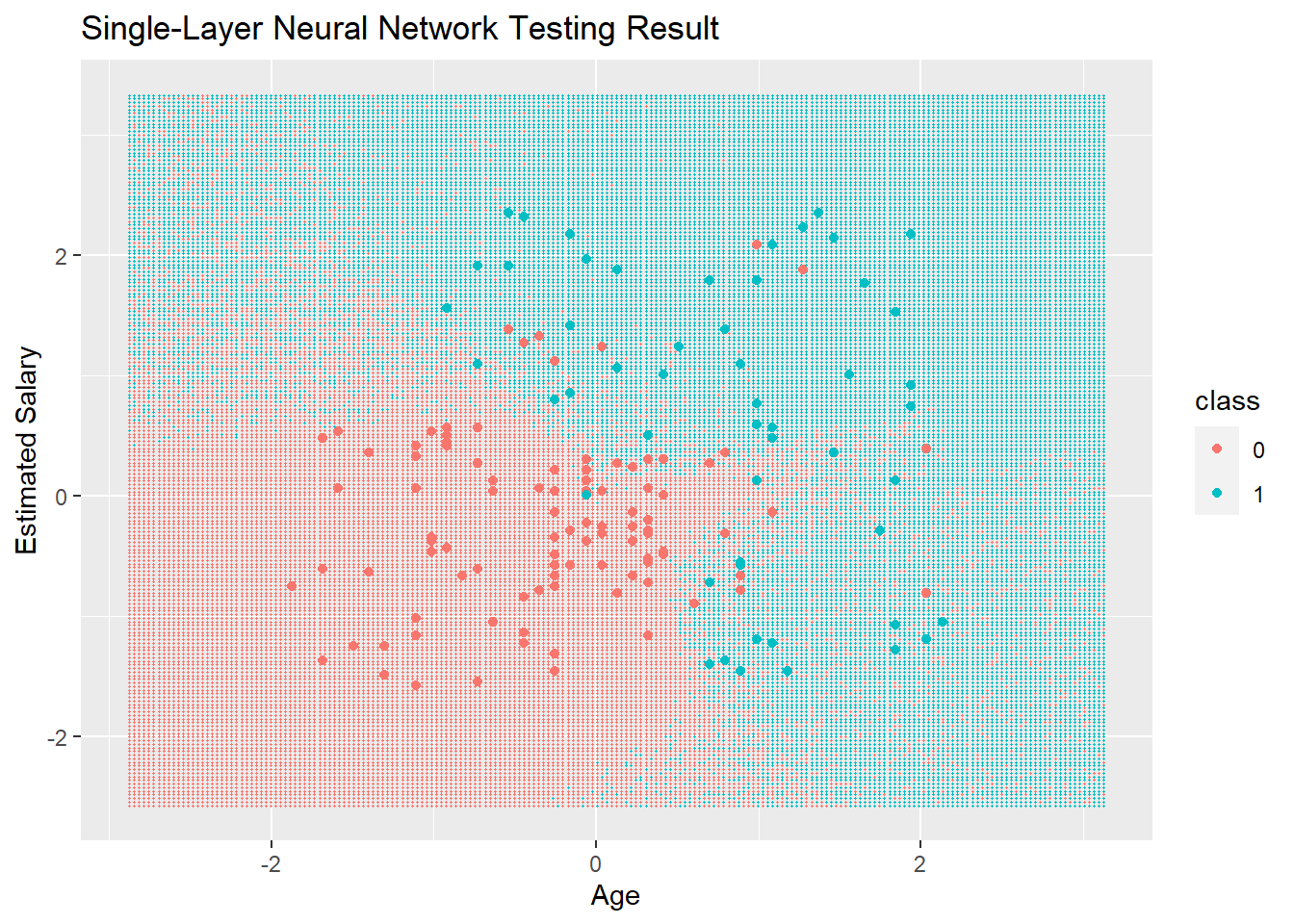options(warn = oldw)

# Process Runtime

end_time <- Sys.time()
end_time - start_time
## Time difference of 57.20434 secs